﻿ Base Space of Nonholonomic System

### Base Space of Nonholonomic System

Tomáš Lipták, Michal Kelemen, Alexander Gmiterko, Ivan Virgala, Ľubica Miková

Journal of Automation and Control

## Base Space of Nonholonomic System

Tomáš Lipták1,, Michal Kelemen1, Alexander Gmiterko1, Ivan Virgala1, Ľubica Miková1

1Department of Mechatronics, Faculty of Mechanical Engineering, Technical University of Košice, Košice, Slovakia

### Abstract

The article deals with the issue of use of geometric mechanics tools at modelling nonholonomic systems. The introductory part of article contains theory of geometric mechanics that we use at creating mathematical model of nonholonomic locomotion system with undulatory movement. Further it contains the determination of reconstruction equation for three-link snake-like robot where we consider ideal source of velocity. The relation between changes of shape and position variables was expressed using the local connection. After determination of controllability of kinematic snake, in last part we created reduced base dynamic equations in case when base variables do not represent ideal source of velocity.

• Tomáš Lipták, Michal Kelemen, Alexander Gmiterko, Ivan Virgala, Ľubica Miková. Base Space of Nonholonomic System. Journal of Automation and Control. Vol. 4, No. 2, 2016, pp 10-14. http://pubs.sciepub.com/automation/4/2/1
• Lipták, Tomáš, et al. "Base Space of Nonholonomic System." Journal of Automation and Control 4.2 (2016): 10-14.
• Lipták, T. , Kelemen, M. , Gmiterko, A. , Virgala, I. , & Miková, Ľ. (2016). Base Space of Nonholonomic System. Journal of Automation and Control, 4(2), 10-14.
• Lipták, Tomáš, Michal Kelemen, Alexander Gmiterko, Ivan Virgala, and Ľubica Miková. "Base Space of Nonholonomic System." Journal of Automation and Control 4, no. 2 (2016): 10-14.

 Import into BibTeX Import into EndNote Import into RefMan Import into RefWorks

123
Prev Next

### 1. Introduction

Classical treatment of multibody system dynamics usually leads to long and complicated equations of motion in case when these equations are expressed in scalar form. These equations are often not suitable for the analysis and design of control. Recent development in geometric mechanics provides a powerful tool for the formulation of equations of motion and understanding of the important features of their dynamics. These results can be summarized in the following points:

1. dynamics of multibody system is independent of certain base configurations, which are defined using group variables - this feature is called as symmetry,

2. for mechanical system with symmetries is possible to obtain simplified equations of motion by removing these invariances - this process is called as reduction,

3. the separation of group and base variables has many advantages at formulation of equations, investigation of dynamic properties and at analysis and design of control.

An important consequence of symmetries is the existence of conserved quantities, e.g. momentum or energy, according to Noether’s theorem. These conserved quantities, described by algebraic equations, are useful to study dynamics and control problems for multibody systems. On the one hand we can use of these quantities to obtain a smaller number of equations, thus simplifying the equations of motion and on the other hand, these quantities impose constraints on system states. This is crucial to recognize in controllability analysis.

Imitation of snake-like locomotion in robotics is an important area of research with respect to high stability and good terrainability compared with wheeled and legged robots. Significant is also their robustness to mechanical failure due to high redundancy. Motion patterns of biological snakes therefore serve as a source of inspiration for snake-like locomotion. To be able to analyze these motion patterns, suitable mathematical models are need. In general, based on observing animal locomotion in nature such as walking of horses or swimming of fishes is possible to find out that the locomotion arises due to change of body shape and its interaction with the environment (Figure 1). This fact is the basis for creating models of robot locomotion.Download asVeiw figureFigures index
Figure 1. Principle of locomotion

In this article we deals with modelling and analysis of flat surface locomotion with sideslip constraints, where the principle of modeling is based on the assumption, that snake body cannot perform a lateral movement, it follows that, it is necessary to establish to motion equations of snake-like robot nonholonomic constraints first kind. In real model of robot we obtain these nonholonomic constraints using wheels. This fact at biological snake is explained by anisotropy of skin friction and surface irregularity on which the snake moves . For creating model of locomotion we inspired by works [2, 3, 4, 5], in which authors tried to research basic mathematical structures that are common for all locomotion systems.

### 2. Geometric Mechanics

Classical mechanics, one of the oldest branches of science, has undergone a long evolution, developing hand in hand with many areas of mathematics, including calculus, differential geometry and the theory of Lie groups and Lie algebras. The modern formulations of Lagrangian and Hamiltonian mechanics, in the coordinate-free language of differential geometry, are elegant and general. They provide a unifying framework for many seemingly disparate physical systems, such as n-particle systems, rigid bodies, fluids and other continua, and electromagnetic and quantum systems .

The modern formulations of Lagrangian and Hamiltonian mechanics use the language of differential geometry. Some advantages of this approach are:

1. it applies to systems on general manifolds, including configuration spaces defined by constraints,

2. it is coordinate-free, or at least independent of a particular choice of coordinates,

3. the geometrical structures have analogues in infinite-dimensional systems.

Just as importantly, the geometric approach provides an elegant and suggestive viewpoint. For example, rigid body motion can be seen as geodesic motion on the rotation group. Symmetries of mechanical systems are represented mathematically by Lie group actions. The presence of symmetry allows a reduction in the number of dimensions of a mechanical system, in two basic ways: by grouping together equivalent states and by exploiting conserved quantities (momentum maps) associated with the symmetry .

### 3. Reconstruction Equation

In following part we will show to create a mathematical model of 3-link kinematic snake and we will consider situation when the base variables represent ideal source of velocity.Download asVeiw figureFigures index
Figure 2. Mathematical model expressed only using reconstruction equation

The undulatory locomotion of 3-link kinematic snake will be provided by modifying the angle size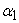,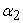, where wheel axes are rigidly held perpendicular to the links (Figure 3). The position and orientation of kinematic snake is represented in the plane by three fiber variables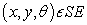(2) and its configuration space is: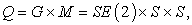(1)

where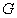is called as fiber space and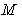as base space.

Three-link kinematic snake has three degrees of freedom given by variables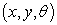and 2 shape (control) variables,. This means, that the snake-like robot belongs to under-actuated nonholonomic mechanical systems first order.Download asVeiw figureFigures index
Figure 3. Kinematic scheme and 3D model of 3-link kinematic snake

For this type of snake we have 3 nonholonomic constraints,and thus we get 3 equations of motion and dimension of SE (2) group is also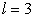, (l=dim{SE (2)}=3). Because of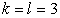three-link kinematic snake belongs to the category of principally kinematic systems, where to build the reconstruction equation fully suffice nonholonomic constraints.

At creating of the mathematical model – reconstruction equation we will use approach where we work with nonholonomic constraints expressed in world coordinates.Download asVeiw figureFigures index
Figure 4. Nonholonomic constraints in world coordinates

For 3-link kinematic snake we have 3 nonholonomic constraints and we will expresses these constraints with respect to coordinate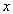and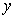: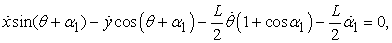(2)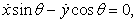(3)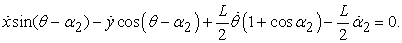(4)

Nonholonomic constraints (2), (3) and (4) we will express in Pfaffian form: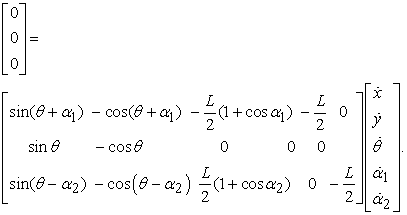(5)

After multiplying of the previous equation and separating group from shape variables we obtain the reconstruction equation in the form: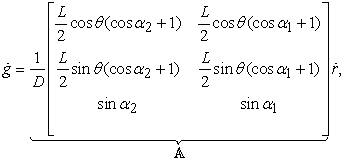(6)

where: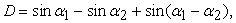and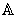represents local connection. Now we express reconstruction equation using local velocities: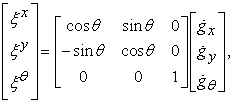(7)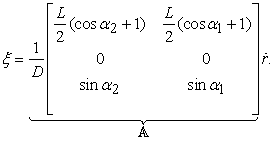(8)

### 4. Controllability of Three-link Kinematic Snake

In this chapter we will investigate controllability of 3-link kinematic snake. First we determine vector fields from mathematical model, equation (6), i.e. we write individual components of local connection: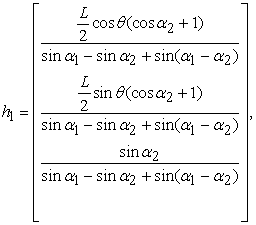(9)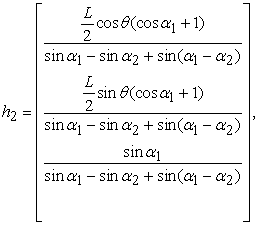(10)

and because of that we have only two vector fields, we can create by their combination only one other vector field. The third vector field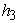will be created using Lie bracket :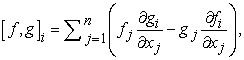(11)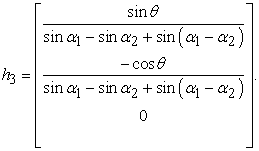(12)

Now we create sum of vector fields: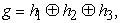(13)

and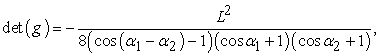(14)

which means that we obtain three independent vector fields, where the number of vector fields is equal to the number of group variables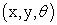i.e. 3-link kinematic snake is locally fiber controllable.

In the case of singular configuration these conditions of controllability do not apply and these areas we determine by equation solution: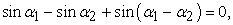(15)

where for chosen= 90°,has two solutions= 180°, 90° and in these configurations kinematic snake are not able to change configuration without breaking nonholonomic constraint.Download asVeiw figureFigures index
Figure 5. Singular configurations of 3-link kinematic snake

In the following figure is shown the serpentine movement of 3-link kinematic snake simulated in program Solid Works 2015 during one period (2π s), where shape variables change according to equation: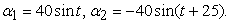(16)Download asVeiw figureFigures index
Figure 6. Lateral movement of 3-link kinematic snake with shown trajectory of center of mass

Travelled distance of snake at serpentine movement during one period is 28.36 mm.

### 5. Reduced base Dynamic Equation

In this chapter we will consider situation where the base variables do not represent ideal source of velocity. The three-link snake will be controlled using torque and so we have to use also reduced base dynamic equation, whose solution are base-shape variables. Then these base variables we substitute to reconstruction equation and obtain fiber velocity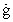or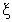.Download asVeiw figureFigures index
Figure 7. Mathematical model expressed using reduced base dynamic equation and reconstruction equation

In general reduced base dynamic equation has form :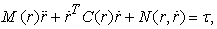(17)

whereis reduced mass-inertia matrix,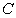contains Coriolis and centripetal force terms and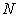includes conservative forces and any additional external forces such as friction.

For creation of reduced base dynamic equation we use Lagrangian, i.e.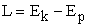, which will be expressed only using kinetic energy, because potential energy is equal to zero, and it is because of that we investigate the movement of 3-link kinematic snake in the plane. Lagrangian for 3-link kinematic snake has form: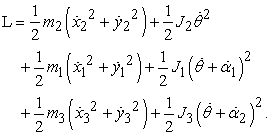(18)

Now we accede to Lagrangian simplification, that is, the kinetic energy for translational and rotational motion can be expressed considering to center of mass of kinematic snake, provided that shape variables we lock in, thus shape variables will be equal zero. We add the kinetic energy of joints to these components. Using m we refer total weight of kinematic snake including the wheels and J will be moment of inertia of kinematic snake around the center of mass. The simplified Lagrangian has form: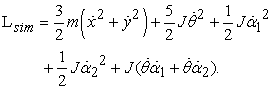(19)

Now we compare course of Lagrangian and simplified Lagrangian during half-period, where shape variables change according to equation (16). Weight, moment of inertia and length were determined using SolidWorks, where model of kinematic snake was created:

• m = 0.05225 kg,

• J = 0.00007487727 kgm2,

• l = 0.16 m.Download asVeiw figureFigures index
Figure 8. Comparison of Lagrangian courses

Differences between courses of Lagrangian were minimum and therefore we will work with the case of simplified Lagrangian, which will greatly simplify our calculations. Form of simplified Lagrangian is invariant and this special form is called as reduced Lagrangian l. This reduced Lagrangian is depend only on body velocities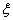and shape variables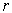,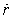and we can express in matrix form: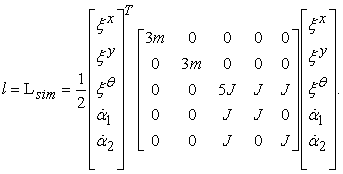(20)

Then we use relation to determine of reduced mass-inertia matrix :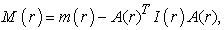(21)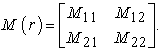(22)

Term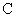is defined by: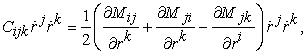(23)

andfor our kinematic snake has form: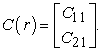(24)

The last termwe determine according to relation: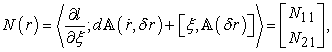(25)

where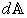is exterior derivative of connection defined as: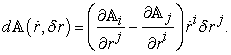(26)

After substituting individual components of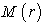,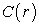and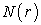to equation (17) we get reduced base dynamic equations in general form: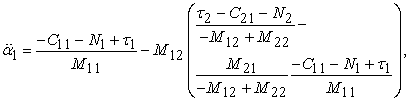(27)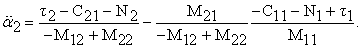(28)

When we will integrate previous equations (27), (28) and base velocities substitute to equation (8), we get desired body velocities of snake center of mass as shown above in Figure 7.

In the following figure we can see difference between courses of accelerations when we consider all components of reduced base dynamic equations,andand situation when we consider only term.Download asVeiw figureFigures index
Figure 9. Comparison of acceleration courses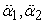with all terms M(r), C(r) and N(r) and only with term M(r)

### 6. Conclusion

The aim of paper was to introduce the use the geometric mechanics at modelling nonholonomic locomotion systems. At first we discussed about advantages of modelling process based on geometric mechanics that we used to create mathematic model of locomotion systems. We deduced mathematic model that is called as reconstruction equation. Detailed process of creating reconstruction equation was presented on three-link kinematic snake. We determined controllability of kinematic snake and found out when singular configurations arise. We solved also reduced base dynamic equations when we control kinematic snake using torques. The main advantage of form of these created reconstruction equations is existence of connection. Individual components of connection we can show using vector fields and then we can analyse the shape of vector fields to the shape of gait by which we obtain the ability to predict how designed locomotion system will move in the plane.

### Acknowledgements

The work has been accomplished under the research project No. VEGA 1/0872/16 “Research of synthetic and biological inspired locomotion of mechatronic systems in rugged terrain”.

### References

  HU D., NIRODY J., SCOTT T., SHELEY M.: The mechanics of slithering locomotion, Proceedings of the National Academy of Sciences, USA 106 (2009), pp. 10081-10085.In article View Article  PubMed  SHAPARE A., WILCZEK F.: Geometry of self-propulsion at low Reynolds number, Journal of Fluid Mechanics 198 (1989) 557-585.In article View Article  MURRAY R., SASTRY S.: Nonholonomic motion planning: steering using sinusoids, IEEE Transactions on Automatic Control, Jan 1993.In article View Article  KELLY S. D., MURRAY R. M.: Geometric phases and locomotion, Journal of Robotic Systems, vol. 12(6), June 1995, pp. 417-431.In article View Article  OSTROWSKI J.: The Mechanics and Control of Undulatory Robotic Locomotion, The dissertation thesis, California Institute of Technology Pasadena, September 19, 1995.In article  OSTROWSKI J.: Computing reduced equations for robotic systems with constraints and symmetries. In: IEEE Transactions on Robotics and Automation, vol. 15, No. 1, Feb 1999.In article View Article